V1

2023/03/21阅读：35主题：全栈蓝

# 一文快速入门Python

Python 是一种解释型、面向对象、动态数据类型的高级程序设计语言，其开源免费、简单易学、应用场景广阔等优势深受开发者的喜爱。本文将通过简单的阐述带您感受 Python 的魅力，适合有一定编程基础的读者阅读。(针对 Python 3.x 编写)

## 1 Python 入门指南

#### Python 的应用场景

Python 的应用场景包括常见的自动化办公、Python 爬虫、数据分析、人工智能以及 web 开发等，应用场景非常辽阔。

#### 常用的函数

##### print 输出函数
``print("hello world")a = "hello world"print("hello world", a)``

##### input 输入函数
``a = input("输入a")# 输入b并且强制转换为int类型b = int(input("输入b"))``

##### map 函数
``a = [1, 2, 3, 4]b = list(map(str, a))print(a, b, sep="\n")``

##### split 函数

split 函数其实是对字符串的一种分割操作。默认参数是空格，我们可以传递任意字符串作为“刀 🔪”

``a = "1,2,3,4"print(a.split(",")) # 用逗号分割字符串``

``a = list(map(int, input().split()))print(a)``

##### len函数

len是一个计算字符串、列表等数据长度的一个函数，这个一般在遍历字符串或数组时经常会用到。##### range函数

range(a,b,c)返回的是[a,b)区间内以c为间隔的一个range对象，可以使用list函数强制转换为列表对象。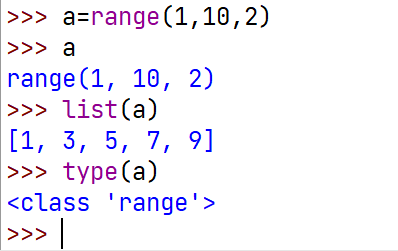## 2 Python 基本操作符

#### Python 注释

Python 的注释有很多种，以下展示了不同注释在 PyCharm 中产生的效果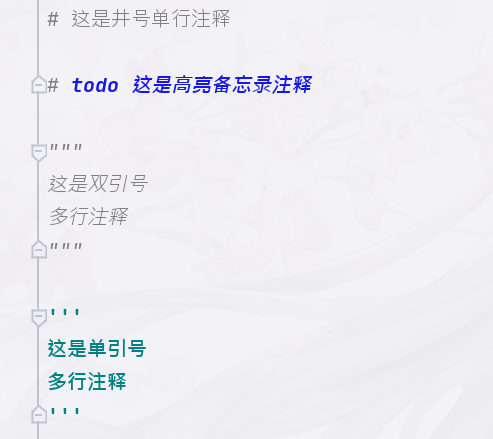其中单引号和双引号是支持多行注释的，井号只支持单行注释。

#### Python 数学运算符

Python 的数学运算符+ - * /等绝大部分都和其他编程语言一致，所以这里只是强调一下特殊的地方。

+= 加等于 x+=1 实质就是 x=x+1，-=;*=都是一个道理
** x=3**4 实质上就是 x=3 的 4 次方
// 取商 9//2 的结果是 4
% 取余 9%2 的结果是 1

#### Python 比较运算符

Python 的比较运算符和大多数编程语言一致，这里要说明的是 Python 不同类型的数据不能使用`>` `<` `>=` `<=`来进行比较，却可以使用`!=` `==`来比较：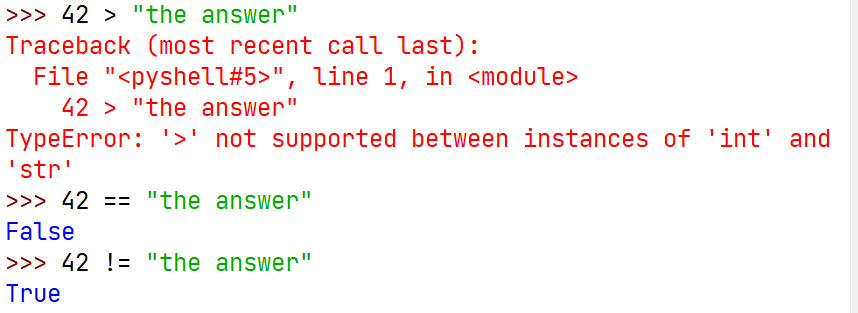除此之外要注意的就是等于号`==`的问题以及浮点数比较涉及的精度问题。

#### Python 成员运算符和身份运算符

`is``not is`表示身份运算符，有点儿类似`==`，但是又不完全相同；实际使用过程中用的还是挺少的，以下是简单的示例：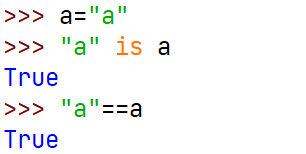#### Python布尔运算符

not x 如果x为True，则返回False，否则返回True
x and y and表示并且，x y同为真时才返回True
x or y or表示或者，x y同为假时才返回False

## 3 Python 基本数据结构

#### 字符串

##### 定义一个字符串
``a = "任何在这双引号之间的文字"b = "单引号其实和双引号完全一样"c = '''三个引号被用于过于长段的文字或者说明，只要三引号不完，你就可以随意换行写下文字'''``
##### 字符串的运算
``a = "你好"b = "很高兴人认识你"print(a + b)  # 字符串相加print(a * 3 + b)  # 字符串与数字相乘print(b[0:3])  # 字符串索引``

##### 字符串格式化符

``a = "小明"b = 1print(f"你好{a}")  # 字符串的格式化print("你好" + a)  # 字符串的加法print(f"你好{b}")  # 字符串与数字的组合``

##### 字符串的一些函数
• split 字符串分割函数，上文已阐述。
• len 字符串长度计算函数，上文已阐述。
• strip 删除字符串两边的空白符（包括：’\n’、’\t’、’\r’）。
• lstrip 删除字符串左边的空白符。
• rstrip 删除字符串右边的空白符。
• upper 将字符串全部变为大写。
• find 查找指定字符在字符串中的位置，如果没有查找到字符，返回-1。
• isdigit 判断字符串是否全由数字组成。
• isalpha 判断字符串是否全由字母组成。
• isalnum 判断字符串是否全由数字或字母组成。

#### 列表

##### 列表的创建
• 普通列表创建，中括号括起来，元素用逗号隔开即可。
``a = [1, 2, "three", "你好"]``
• 列表推导式创建
``b = [i for i in range(10)]c = [i ** 2 for i in range(10)]d = [i for i in range(10) if i % 2 == 0]print(b, c, d, sep="\n")``

输出结果：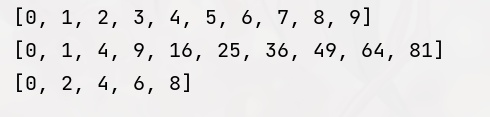##### 操作列表(list)
• len 计算列表的长度，可以配合for循环遍历列表使用。
• append 在列表的末尾添加指定的对象，不返回新的列表，即没有返回值，但是对列表确实做了修改。
• li.insert(a,b) 将b插入到列表li的a位置处。
• pop(a) 将下标为a的元素删除，并返回其对应的元素值。
• remove(a) 将列表中第一个值为a的元素删掉，和pop函数的区别是这个是按值删除，pop函数是按位置删除。
• count(a) 统计列表中a的出现次数。
• li.sort() 将li进行排序。
• list(a) 将可迭代对象a强制转换为列表对象，如果你认真看了前面的内容，你会发现这在接收一组空格分隔的数据存为列表的操作中使用过。

#### 字典(dict)

``# 字典的定义a = {    'one': 1,    'two': 2,    'three': 3}``
##### 字典的一些操作
``# 字典取值print(a['one'])# 字典值的修改a['two'] = "二"print(a)# 添加多个元素a.update({'four': 4, 'five': 5})print(a)``

#### 集合(set)

Python集合在某种意义上来说和数学上的定义很相似，但是不能被切片和索引，作者也很少用到集合，一般都是利用它的值的唯一性，来去除列表中的重复元素：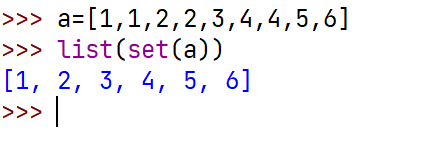## 4 Python 选择语句

Python中的选择语句就只有if和elif,else三个关键词，搭配前面学到的逻辑判断，Python的选择结构，包括后面的循环结构，条件语句都是不需要使用括号的，这一点和绝大多数编程语言都不相同。

``a = 1b = 2if a == 1 and b != 2:    print("a,b", a, b)else:    print("b,a", b, a)``

## 5 Python 循环语句

#### for 循环

for循环一般和range函数一起并用，通常可以用来遍历列表，遍历字符串。

``# 使用for循环遍历字符串的两种方式a = "hello world"for i in a:    print(i, end=" ")print("第二次遍历")for i in range(len(a)):    print(a[i], end=" ")``

``# 使用for循环遍历列表的两种方式a = [str(i) for i in range(10)]for i in a:    print(i, end=" ")print("第二次遍历")for i in range(len(a)):    print(a[i], end=" ")``

#### while循环

`while 条件：`即可进入while循环，使用while循环一定要注意循环是否可以正常退出，保证循环不会进入死循环，否则对硬件会有损伤。

``i = 1while i <= 5:    print(i)    i += 1``

## 6 补充知识

• Python 的语法对空格要求不是很高，只要保证变量或者函数的单词之间没有空格即可，但是对语法缩进有着极其的苛刻要求，因为 Python 的代码块都是基于缩进而产生的。

• Python 的具体下载安装在此就不强调，可以参考作者的 CSDN 文章-听说你还不会 python 环境的安装与配置？_小 y 同 学的博客-CSDN 博客 里面详细介绍了 Python 的安装以及一些 PyCharm 使用技巧。

• Python 安装完成之后 一般都会产生如下快捷方式，很多初学者包括作者都不知到其具体的作用，在此可以跟大家简单科普一下：第一个 IDLE 是 Python 自带的一个交互式工具，所谓交互式就可以简单理解聊天式，打开是一个白色界面，作者一般都会把他固定到任务栏偶尔作为计算器使用。第二个 Python 是命令行的交互式，和我们直接在命令行窗口敲 Python 所产生的效果一致。第三个算是 Python 的一个帮助文档。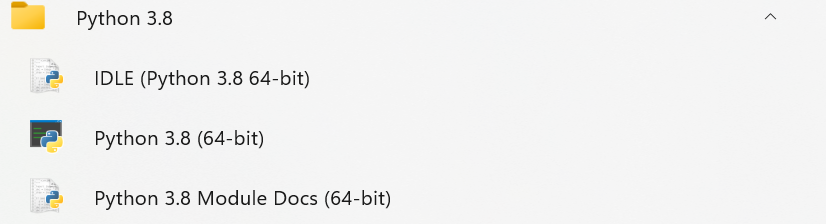• Python 的字符串可以使用单引号，也可以使用双引号，这一点完全看个人喜好和心情。

• Python 列表、字典等大多数数据类型都是可以直接打印的，没有必要像 C 语言那样还得写个循环才能打印出数组的元素。

• 路虽远，行则将至；事虽难，做则必成。希望认真学习的你能够有所收获！

• 本公众号的原创成果，在未经允许的情况下，请勿用于任何商业用途！

• 您的点赞、收藏与关注是我创作的最大动力！